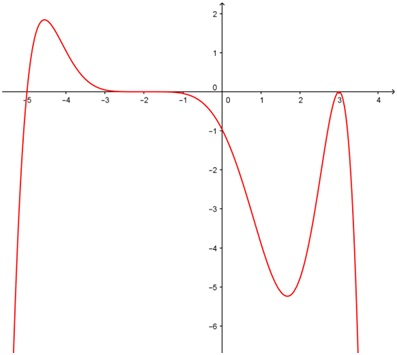Multiplicities of polynomials

Multiplicities of polynomials

Lessons

The multiplicity of a zero corresponds to the number of times a factor is repeated in the function.
$\cdot$ Odd multiplicity: cross the x-axis
$\cdot$ Odd multiplicity (3 or more): changes concavity when passing through x-axis
$\cdot$ Even multiplicity: bounces off the x-axis
• 1.
Sketch the graph of the polynomial function.
i)
$P\left( x \right) = x - 2$
ii)
$P\left( x \right) = {\left( {x - 2} \right)^2}$
iii)
$P\left( x \right) = {\left( {x - 2} \right)^3}$
iv)
$P\left( x \right) = {\left( {x - 2} \right)^4}$
v)
$P\left( x \right) = {\left( {x - 2} \right)^5}$

• 2.
Given that the graph shows a degree-eight polynomial and the zero $x = 3$ has a multiplicity of 2, determine the multiplicity of the zero $x = - 2$.• 3.
Without using a graphing calculator, make a rough sketch of the following polynomial: $P\left( x \right) = - \frac{1}{{12907776}}\left( {x + 3} \right){\left( {x + 1} \right)^2}{\left( {x - 2} \right)^3}{\left( {x - 4} \right)^4}{\left( {x - 7} \right)^5}$